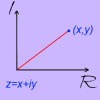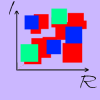# Complex Numbers Further### A Brief Introduction to Complex Numbers

##### Age 14 to 18Challenge Level

In this problem, we define complex numbers and invite you to explore what happens when you add and multiply them.### A Brief Introduction to the Argand Diagram

##### Age 14 to 18Challenge Level

Complex numbers can be represented graphically using an Argand diagram. This problem explains more...### Complex Squares

##### Age 16 to 18Challenge Level

What happens when we square complex numbers? Can the square of a complex number be real?### Thousand Words

##### Age 16 to 18Challenge Level

Here the diagram says it all. Can you find the diagram?